# Gas consumption

The vessel consume 100 tons of gas in 250 miles. How many fuel will the vessel consume if it travels 400 miles?

Result

x =  160 t

#### Solution:Leave us a comment of example and its solution (i.e. if it is still somewhat unclear...):

Showing 0 comments:Be the first to comment!#### To solve this example are needed these knowledge from mathematics:

Tip: Our volume units converter will help you with converion of volume units.

## Next similar examples:

1. Gasoline canisters35 liters of gasoline is to be divided into 4 canisters so that in the third canister will have 5 liters less than in the first canister, the fourth canister 10 liters more than the third canister and the second canister half of that in the first canist
2. Potatoes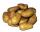Potatoes contain 78.6% starch. How many potatoes need to obtain 27 kg of starch?
3. Fish tankA fish tank at a pet store has 8 zebra fish. In how many different ways can George choose 2 zebra fish to buy?
4. Cows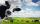4 cows spent 16 bags of hay in 5 days. How many bags of hay sacks is needed for 5 cows for seven days?
5. RunnersFor three runners (on the first to third place) is prepared 30 chocolate that they be distributed in the ratio of 3 : 2 : 1 How much chocolate will get everyone?
6. Theorem proveWe want to prove the sentense: If the natural number n is divisible by six, then n is divisible by three. From what assumption we started?
7. SequenceFind the common ratio of the sequence -3, -1.5, -0.75, -0.375, -0.1875. Ratio write as decimal number rounded to tenth.
8. Cross-sections of a coneCone with base radius 16 cm and height 11 cm divide by parallel planes to base into three bodies. The planes divide the height of the cone into three equal parts. Determine the volume ratio of the maximum and minimum of the resulting body.
9. Numbers at ratio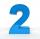The two numbers are in a ratio 3:2. If we each increase by 5 would be at a ratio of 4:3. What is the sum of original numbers?
10. Barrel with water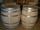The water barrel has a weight of 52 kg. When we pour a quarter of the water, the weight of the barrel is reduced to 40 kg. Determine the weight of the empty barrel.
11. Gain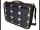Find the gain whenever the output power is 21x the input power.
12. Train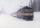The train passes part of the line for 95 minutes at speed 75 km/h. What speed would have to go in order to shorten the driving time of 20 minutes?
13. Sequence 3Write the first 5 members of an arithmetic sequence: a4=-35, a11=-105.
14. Linear independence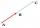Determine if vectors u=(-4; -5) and v=(20; 25) are linear Linear dependent.
15. Complex number coordinatesWhich coordinates show the location of -2+3i
16. Six termsFind the first six terms of the sequence a1 = -3, an = 2 * an-1
17. EquationEquation ? has one root x1 = 8. Determine the coefficient b and the second root x2.